# AP Physics 1 Multiple-Choice Practice Test 33

### Test Information10 questions18 minutes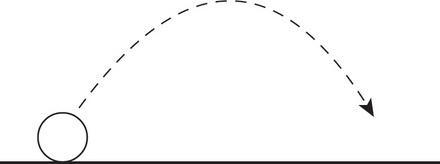1. Which of the following is a true statement regarding the motion of projectiles?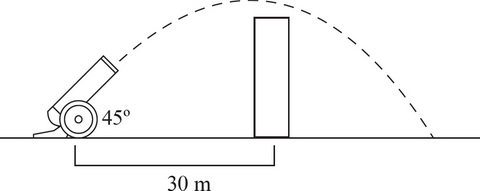2. A cannonball is fired with an initial velocity of 20 m/s and a launch angle of 45° at a wall 30 m away. If the cannonball just barely clears the wall, what is the maximum height of the wall?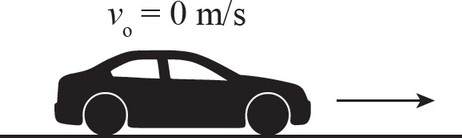3. A car initially at rest accelerates linearly at a constant rate for 5 s. If the final speed of the car is 15 m/s, what was the displacement of the car?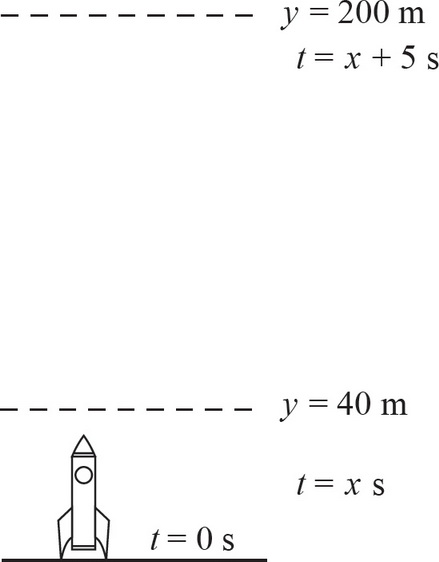4. A rocket is launched into the air. A few moments after liftoff, the rocket is 40 m above the ground. After another 5 s, the rocket is now 200 m off the ground. What is the average velocity of the rocket during the 5 s part of the flight?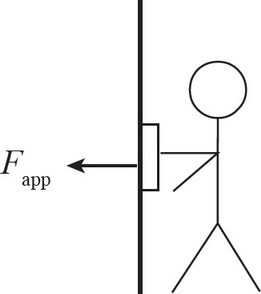5. A student presses a 0.5 kg book against the wall. If the µs between the book and the wall is 0.2, what force must the student apply to hold the book in place?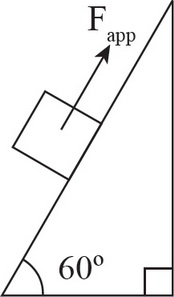6. A box with a mass of 10 kg is placed on an inclined plane that makes a 60° angle with the horizontal. The coefficient of static friction (μs) between the box and the inclined plane is 0.2. What force must be applied on the box in order to prevent the box from sliding down the inclined plane?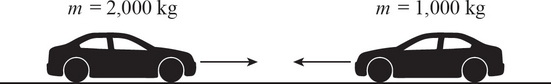7. A 2,000 kg car has a head-on collision with a 1,000 kg car. How does the impact force on the heavier car compare with that of the smaller car?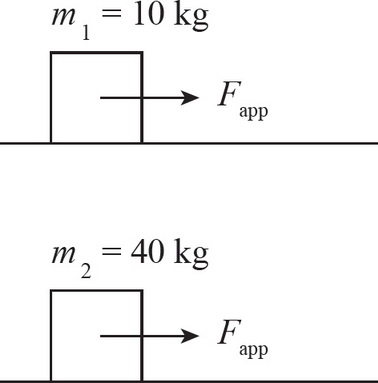8. Two students push two different boxes across a frictionless floor. Both students exert a horizontal force of 50 N. If the first box has mass m1 = 10 kg and the second box has mass m2 = 40 kg, what is the value of a1/a2?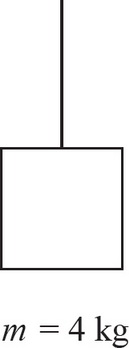9. A 4 kg box is accelerated upwards by a string with a breaking strength of 80 N. What is the maximum upward acceleration that can be applied on the box without breaking the string?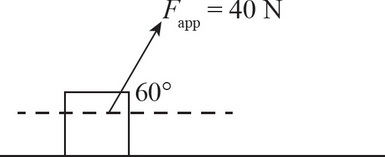10. A worker moves a 30 kg box by pulling on it with a rope that makes a 60° angle with the horizontal. If the worker applies a force of 40 N and pulls the box over a distance of 20 m, how much work did the worker do?